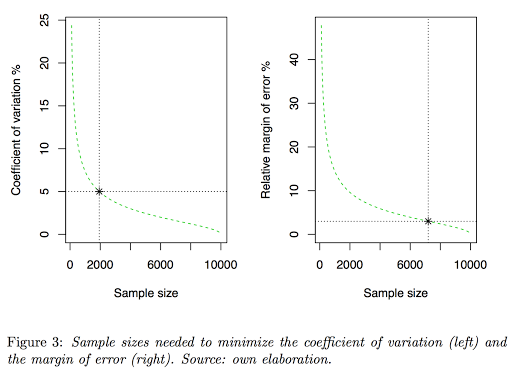[This article was first published on Data Literacy - The blog of Andrés Gutiérrez, and kindly contributed to R-bloggers]. (You can report issue about the content on this page here)
Want to share your content on R-bloggers? click here if you have a blog, or here if you don't.

The R package samplesize4surveys contains functions that allow to calculate sample sizes for estimating proportions, means, difference of proportions and even difference of two means. It also permits the calculation of sample error and power level for a fixed sample size.

Here four functions are introduced for the estimation of a population variance and for conducting statistical hypothesis testing on this parameter of interest. Right away is the description of these functions:

1. Function ss4S2 allows calculating the sample size for estimating \$s^2_{y_U}\$ subject to a particular value of the coefficient of variation or the relative margin of error. Additionally, it offers to the user the option of mapping the coefficient of variation and the margin of error as a function of the sample size, to make easier the decision about \$n\$.
2. Function ss4S2H allows calculating the sample size for estimating \$s^2_{y_U}\$ subject to a particular power level to detect a population variance greater than the value set in the null hypothesis. It also offers to the user the option of mapping the power level in function of the sample size.
3. Function e4S2 allows calculating the coefficient of variation and the margin of error for a particular sample size. It also allows obtaining a mapping similar to the one of ss4S2.
4. Function b4S2 allow calculating the power level for a fixed sample size. It also allows obtaining a mapping similar to the one of ss4S2H

In order to use the above functions it is necessary to install and call the package that contains them in the Comprehensive R Archive Network (CRAN). That for, it is required to type the following code lines from the console:

```install.packages("samplesize4surveys")
library(samplesize4surveys)
```

For example, the following code line gives the necessary sample size to estimate the variance of a characteristic of interest in a finite population (with a coefficient of kurtosis of one) to reach an estimated coefficient of variation of maximum 5% and a relative margin of error of 3%

```ss4S2(N = 10000, K = 1, CV = 0.05, me = 0.03, DEFF = 2, plot = TRUE)

```On the other hand, as the package is in constant update, the authors have arranged a repository in which users can use the newest features and interact with the academic community to correct possible errors in computer codes and improve the efficiency of functions, among others. In order to access to this version control, it is necessary to type the following lines from R.

```library(devtools)
install_github("psirusteam/samplesize4surveys")```

In this paper you can find the mathematical background behind those R functions.